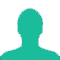# In order to tell Excel that we are entering a formula in cell, we must begin with an operator such as

A. \$

B. @

C. +

D. =

E. #

### Solution(By Examveda Team)

In MS Excel, formulas are equations that perform various calculations in your worksheets. Though Microsoft has introduced a handful of new functions over the years, the concept of Excel spreadsheet formulas is the same in all versions of Excel 2016, Excel 2013, Excel 2010, Excel 2007 and lower.
All Excel formulas begin with an equal sign (=).

1.Plz

2.Which

3.The file extension of MS. World document in Office 2010 is ______.
A.*.doc
B.*.docx
C.*.pdf
D*.txt

4.Which of the following memory is non-volatile?
A.SRAM
B.DRAM
C.ROM
D.ALL OF THE ABOVE

5.it may be + in some cases.

Related Questions on Computer Fundamental Miscellaneous